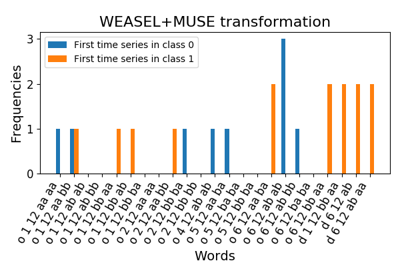# `pyts.multivariate.transformation`.WEASELMUSE¶

class `pyts.multivariate.transformation.``WEASELMUSE`(word_size=4, n_bins=4, window_sizes=[0.1, 0.3, 0.5, 0.7, 0.9], window_steps=None, anova=False, drop_sum=True, norm_mean=True, norm_std=True, strategy='quantile', chi2_threshold=2, sparse=True, alphabet=None)[source]

WEASEL+MUSE algorithm.

Parameters: word_size : int (default = 4) Size of each word. n_bins : int (default = 4) The number of bins to produce. It must be between 2 and 26. window_sizes : array-like (default = [0.1, 0.3, 0.5, 0.7, 0.9]) Size of the sliding windows. All the elements must be either integers or floats. In the latter case, each element represents the percentage of the size of each time series and must be between 0 and 1; the size of the sliding windows will be computed as `np.ceil(window_sizes * n_timestamps)`. window_steps : None or array-like (default = None) Step of the sliding windows. If None, each `window_step` is equal to `window_size` so that the windows are non-overlapping. Otherwise, all the elements must be either integers or floats. In the latter case, each element represents the percentage of the size of each time series and must be between 0 and 1; the step of the sliding windows will be computed as `np.ceil(window_steps * n_timestamps)`. anova : bool (default = False) If True, the Fourier coefficient selection is done via a one-way ANOVA test. If False, the first Fourier coefficients are selected. drop_sum : bool (default = True) If True, the first Fourier coefficient (i.e. the sum of the subseries) is dropped. Otherwise, it is kept. norm_mean : bool (default = True) If True, center each subseries before scaling. norm_std : bool (default = True) If True, scale each subseries to unit variance. strategy : str (default = ‘quantile’) Strategy used to define the widths of the bins: ‘uniform’: All bins in each sample have identical widths ‘quantile’: All bins in each sample have the same number of points ‘normal’: Bin edges are quantiles from a standard normal distribution ‘entropy’: Bin edges are computed using information gain chi2_threshold : int or float (default = 2) The threshold used to perform feature selection. Only the words with a chi2 statistic above this threshold will be kept. sparse : bool (default = True) Return a sparse matrix if True, else return an array. alphabet : None, ‘ordinal’ or array-like, shape = (n_bins,) Alphabet to use. If None, the first n_bins letters of the Latin alphabet are used.

References

 [Rdb6c83bc7051-1] P. Schäfer, and U. Leser, “Multivariate Time Series Classification with WEASEL+MUSE”. Proceedings of ACM Conference, (2017).

Examples

```>>> from pyts.datasets import load_basic_motions
>>> from pyts.multivariate.transformation import WEASELMUSE
>>> X_train, X_test, y_train, y_test = load_basic_motions(return_X_y=True)
>>> transformer = WEASELMUSE()
>>> X_new = transformer.fit_transform(X_train, y_train)
>>> X_new.shape
(40, 9086)
```
Attributes: vocabulary_ : dict A mapping of features indices to terms. Each value is a string with 4 values separated by a whitespace: ‘o’ or ‘d’: whether the word is extracted from the original time series (‘orig’) or from the derivates (‘diff’) int : feature index int : window size str : word

Methods

 `__init__`(self[, word_size, n_bins, …]) Initialize self. `fit`(self, X, y) Fit the model according to the given training data. `fit_transform`(self, X, y) Fit the data then transform it. `get_params`(self[, deep]) Get parameters for this estimator. `set_params`(self, \*\*params) Set the parameters of this estimator. `transform`(self, X) Transform the provided data.
`__init__`(self, word_size=4, n_bins=4, window_sizes=[0.1, 0.3, 0.5, 0.7, 0.9], window_steps=None, anova=False, drop_sum=True, norm_mean=True, norm_std=True, strategy='quantile', chi2_threshold=2, sparse=True, alphabet=None)[source]

Initialize self. See help(type(self)) for accurate signature.

`fit`(self, X, y)[source]

Fit the model according to the given training data.

X : array-like, shape = (n_samples, n_features, n_timestamps)
Multivariate time series.
y : array-like, shape = (n_samples,)
Class labels.
Returns: self : object
`fit_transform`(self, X, y)[source]

Fit the data then transform it.

X : array-like, shape = (n_samples, n_features, n_timestamps)
Multivariate time series.
y : array-like, shape = (n_samples,)
Class labels.
Returns: X_new : array, shape = (n_samples, n_features_new) Document-term matrix with relevant features only.
`get_params`(self, deep=True)

Get parameters for this estimator.

Parameters: deep : bool, default=True If True, will return the parameters for this estimator and contained subobjects that are estimators. params : mapping of string to any Parameter names mapped to their values.
`set_params`(self, **params)

Set the parameters of this estimator.

The method works on simple estimators as well as on nested objects (such as pipelines). The latter have parameters of the form `<component>__<parameter>` so that it’s possible to update each component of a nested object.

Parameters: **params : dict Estimator parameters. self : object Estimator instance.
`transform`(self, X)[source]

Transform the provided data.

Parameters: X : array-like, shape = (n_samples, n_features, n_timestamps) Multivariate time series. X_new : sparse matrix, shape = (n_samples, n_features_new) Document-term matrix with relevant learned features only.

## Examples using `pyts.multivariate.transformation.WEASELMUSE`¶WEASEL+MUSE